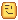# Train node system

I want to make a train system that is based on nodes.
Just like StepfordCountyRailway

But not numbered nodes, the train should recognize the next node in front of it to travel to and then Tween to it. After that the other carriages should follow the carriage infront of it.

As of now, I currently cant get the train to have the same distance when you change the speed.
It will either increase the speed or decrease it making it not a functional train.

Code StarterCharacterScripts
``````for i, track in pairs(game.Workspace:WaitForChild("Track"):GetChildren()) do
if track then
for i, node in pairs(track:WaitForChild("Nodes"):GetChildren()) do
node.Parent = game.Workspace.Node
end
end
end
local TweenService = game:GetService("TweenService")

--local Body_val = #workspace.Train:GetChildren() - 1

a = {}
local numn = #workspace.Node:GetChildren()

local last = math.huge
local part
local check=0
for i,v in pairs(workspace.Node:GetChildren()) do
if a[v]==true then
check+=1
end
end
if check == numn then
a = {}
end
for i,v in pairs(workspace.Node:GetChildren()) do
local m = (Train_Head.Position - v.Position).magnitude
if not a[v] == true and m < last then
last = m
part = v
end
end
return part
end

for i, train in pairs(game.Workspace.Trains:GetChildren()) do
local Body_val = (#train.Carriages:GetChildren()*2) +1
if #a < numn then
local distance = (Train_head1.CFrame.Position - part.CFrame.Position).Magnitude
local speed = train.Setting.Speed2.Value-- studs/s
local t = distance/speed
local distance2 = (train.Carriages:GetChildren().NotAnchor.FirstBoogiePart.CFrame.Position - train.Head.NotAnchor.FirstBoogiePart.CFrame.Position).Magnitude
print(distance2)
print(distance3)
local bd = 7.8-t
local cd = 15-t
--train.Setting.TrainDistance.Value = cd
--train.Setting.BoogieDistance.Value = bd
warn(bd)
warn("|| "..cd)
--local t = train.Setting.Speed.Value
local twin4 = TweenInfo.new(t, Enum.EasingStyle.Linear, Enum.EasingDirection.In,0, false,0)
local togo = part.CFrame
a[part] = true
TweenService:Create(Train_head1,twin4,{CFrame = togo * CFrame.new(0,1.85,0) }):Play()
delay(wait(),function()
wait(train.Setting.BoogieDistance.Value)
TweenService:Create(Train_head2,twin4,{CFrame = togo * CFrame.new(0,1.8,0) }):Play()
end)

delay(wait(),function()
warn(train.Setting.TrainDistance.Value)
for i, coach in pairs(train.Carriages:GetChildren()) do

TweenService:Create(coach.FirstBoogie.Main,twin4,{CFrame = togo * CFrame.new(0,1.8,0)}):Play()
delay(wait(),function()
wait(train.Setting.BoogieDistance.Value)
TweenService:Create(coach.LastBoogie.Main,twin4,{CFrame = togo * CFrame.new(0,1.8,0)}):Play()
end)
wait(train.Setting.TrainDistance.Value)
--local thisbody = 1
--if Body_val == 1 then
--	TweenService:Create(workspace.Train["Body"..1],twin4,{CFrame = togo * CFrame.new(0,1,0)}):Play()
--elseif Body_val >= 2 then
--	for i = 1, Body_val do
--		TweenService:Create(workspace.Train["Body"..thisbody],twin4,{CFrame = togo * CFrame.new(0,1,0)}):Play()
--		thisbody+=1
--		wait(train.Setting.TrainDistance.Value)
--	end
--end
end
end)

wait(t)
else

a = {}

end
end

end```
``````

Im sorry if I couldnt explain the issue clearly!

Thank you in advance!3 Likes

ok so you want to make a nodes so what you can do is get the magnitude of the node but the problem is if you are making curves then it will jut move on without following the curve which is not ideal in your case. But you can still do one thing. You can rotate the nodes and make a loop to check the nearest node and its rotation relative to the trains engines rotation. So

``````local Nodes = YourFolderDirectory
local Train = YourTrainDirectory
local NearNode = nil
function GetNearestNode()
for i,v in ipairs(Nodes:GetChildren()) do
if NearNode ~= nil then
if NearNode.Magnitude > v.Magnitude and NearNode.Orientation.X > v.Orientation.X then
NearNode = v
else
if Train.Magnitude - Node.Magnitude <= YourLimitMustBeANumber then
NearestNode = v
end
end
end)
``````

Though why dont you want to use Indexed Nodes they are just way easier to work with.

i dont think you quite understand my problem, I need the same distance between each carriage or boogie even when changing the speed.

well thats also simple but it requires a bit calculation and it has 2 ways.

1st way : Calculate How much time does it take for your tween to move to a distance equivalent of the boogies length.

2nd Way : weld your train. This is the best way for your case. Why? Its physics based now so it will steer naturally.

So for 1st way you could calculate time by

Dist/Time of tween, Then dividing tht with the length of boogie and waitong for that amount of time and then firing the Tween.
So

``````local WaitTime = BoogieLength/(Magnitude/Time)
for i,v in ipairs(TrainBoogieDirectory:GetChildren())
local NewTween = TweenService:Create(v.PrimaryPart --Assuming its a model
,YourTweenInfoButTimeShouldBeSameForEachTrainAsAbove,YourPropertiesButMagnitudeMustRemainSame)
NewTween:Play()
wait(WaitTime)
``````

For Second Part Just do Welding

I recommend you looking in my code because there is already the Code you described^^ and I wanna use the first method

Ok sorry i didn’t see the code first. But i see the problem that if you will Increase the speed or decrease it, it won’t reflect on the other parts/carriages. So the best thing is to weld them because it will be Irregular if you try the first method.And Don’t worry about it going in a straight line it will steer but you must put the ends together not the whole Part. If I wasn’t able to help much ( or tbh not help at all xD) I’m Sorry for that. But now I shall take my adios. bye and good luck